## Generalized Hypergeometric Function

The generalized hypergeometric function is given by a Hypergeometric Series, i.e., a series for which the ratio of successive terms can be written(1)

(The factor ofin the Denominator is present for historical reasons of notation.) The resulting generalized hypergeometric function is written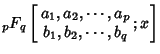(2)(3)

whereis the Pochhammer Symbol or Rising Factorial(4)

If the argument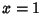, then the function is abbreviated(5)is the'' Hypergeometric Function, andis the Confluent Hypergeometric Function. A function of the formis called a Confluent Hypergeometric Limit Function.

The generalized hypergeometric function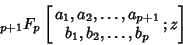(6)

is a solution to the Differential Equation(7)

where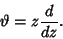(8)

The other linearly independent solution is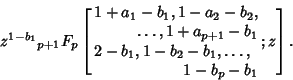(9)

A generalized hypergeometric equation is termed well posed'' if(10)

Many sums can be written as generalized hypergeometric functions by inspection of the ratios of consecutive terms in the generating Hypergeometric Series. For example, for(11)

the ratio of successive terms is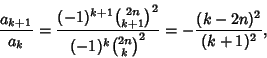(12)

yielding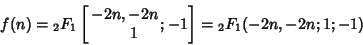(13)

(Petkovsek 1996, pp. 44-45).

Gosper (1978) discovered a slew of unusual hypergeometric function identities, many of which were subsequently proven by Gessel and Stanton (1982). An important generalization of Gosper's technique, called Zeilberger's Algorithm, in turn led to the powerful machinery of the Wilf-Zeilberger Pair (Zeilberger 1990).

Special hypergeometric identities include Gauss's Hypergeometric Theorem(14)

for, Kummer's Formula(15)

whereandis a positive integer, Saalschütz's Theorem(16)

forwith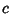a negative integer andthe Pochhammer Symbol, Dixon's Theorem(17)

where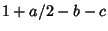has a positive Real Part,, and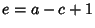, the Clausen Formula(18)

for,,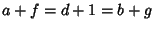,a nonpositive integer, and the Dougall-Ramanujan Identity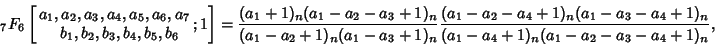(19)

where,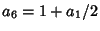,, and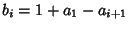for, 2, ..., 6. For all these identities,is the Pochhammer Symbol.

Gessel (1994) found a slew of new identities using Wilf-Zeilberger Pairs, including the following:(20)(21)(22)(23)

(Petkovsek et al. 1996, pp. 135-137).

See also Carlson's Theorem, Clausen Formula, Confluent Hypergeometric Function, Confluent Hypergeometric Limit Function, Dixon's Theorem, Dougall-Ramanujan Identity, Dougall's Theorem, Gosper's Algorithm, Heine Hypergeometric Series, Hypergeometric Function, Hypergeometric Identity, Hypergeometric Series, Jackson's Identity, Kummer's Theorem, Ramanujan's Hypergeometric Identity, Saalschütz's Theorem, Saalschützian, Sister Celine's Method, Thomae's Theorem, Watson's Theorem, Whipple's Transformation, Wilf-Zeilberger Pair, Zeilberger's Algorithm

References

Bailey, W. N. Generalised Hypergeometric Series. Cambridge, England: Cambridge University Press, 1935.

Dwork, B. Generalized Hypergeometric Functions. Oxford, England: Clarendon Press, 1990.

Exton, H. Multiple Hypergeometric Functions and Applications. New York: Wiley, 1976.

Gessel, I. Finding Identities with the WZ Method.'' Theoret. Comput. Sci. To appear.

Gessel, I. and Stanton, D. Strange Evaluations of Hypergeometric Series.'' SIAM J. Math. Anal. 13, 295-308, 1982.

Gosper, R. W. Decision Procedures for Indefinite Hypergeometric Summation.'' Proc. Nat. Acad. Sci. USA 75, 40-42, 1978.

Petkovsek, M.; Wilf, H. S.; and Zeilberger, D. A=B. Wellesley, MA: A. K. Peters, 1996.

Saxena, R. K. and Mathai, A. M. Generalized Hypergeometric Functions with Applications in Statistics and Physical Sciences. New York: Springer-Verlag, 1973.

Slater, L. J. Generalized Hypergeometric Functions. Cambridge, England: Cambridge University Press, 1966.

Zeilberger, D. A Fast Algorithm for Proving Terminating Hypergeometric Series Identities.'' Discrete Math. 80, 207-211, 1990.